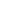# Free CBSE Class 10 Mathematics UNIT IV: Geometry Triangles Worksheets

Download free printable Triangles Worksheets to practice. With thousands of questions available, you can generate as many Triangles Worksheets as you want.

## Sample CBSE Class 10 Mathematics UNIT IV: Geometry Triangles Worksheet Questions

1.

Triangle can be formed using three points.

1. True 2. False
2.

Triangles have four sides.

1. True 2. False
3.

The two sides of a triangle are 6cm and 10cm. What is the maximum possible area of this triangle?

1.

24 cm^2

2.

48 cm^2

3.

60 cm^2

4.

30 cm^2

4.

State True/False: P and Q are the points on the sides DE and DF of a triangle DEF such that DP = 4 cm, DE = 12 cm, DQ= 7 cm and QF = 21 cm. Then .

1.

True

2.

False

5.

Choose the correct option: It is given that , AB = 4 cm, AC = 8 cm and DF= 3 cm. Then DE = ?

1.

3 cm

2.

6 cm

3.

6.

Choose the correct option: It is given that , with BC/ QR =1/2. Then, ar (PRQ)/ ar (BCA) is equal to ________.

1.

1/2

2.

1/8

3.

7.

P and Q are the points on the sides AB and AC of a triangle ABC such that AP = 5 cm, AB = 15 cm, AQ= 6 cm If . Find AC.

1.

18 cm

2.

9 cm

3.

12 cm

4.

8.

ABCD is a trapezium such that and AP = 15, PD = 12, QC = 14, then find BQ?1.

14 cm

2.

7.5 cm

3.

17.5  cm

4.

None of these

9.

In the given figure, PT is the bisector of QPR. Find the perimeter of PQR?1.

21.6  cm

2.

7 cm

3.

43.2  cm

4.

14 cm

10.

In the given figure RP/PK = 3/2. Find ar(TRK) / ar(TPK)?1.

3/2

2.

5/2

3.

3/5

4.

2/5

Worksheets by UrbanPro

Our worksheets are designed to help students explore various topics, practice skills and enrich their subject knowledge, to improve their academic performance. Designed by Experts who have extensive experience and expertise in teaching a subject, these worksheets will improve your child's problem-solving skills and subject knowledge in a fun and interactive manner.
Check out our free customized worksheets across school boards, grades, subjects and levels of subject knowledge. You can download, print and share these worksheets with anyone, anywhere, anytime!

Get a custom worksheet to practice!

Select your topic & see the magic.

subjectSelect Chapter(s)

Chapters & Subtopics